# OCR Maths Papers

Here you will find all the free practice OCR GCSE maths papers and GCSE past papers. Click the links below or use the filtering options to find the papers you need.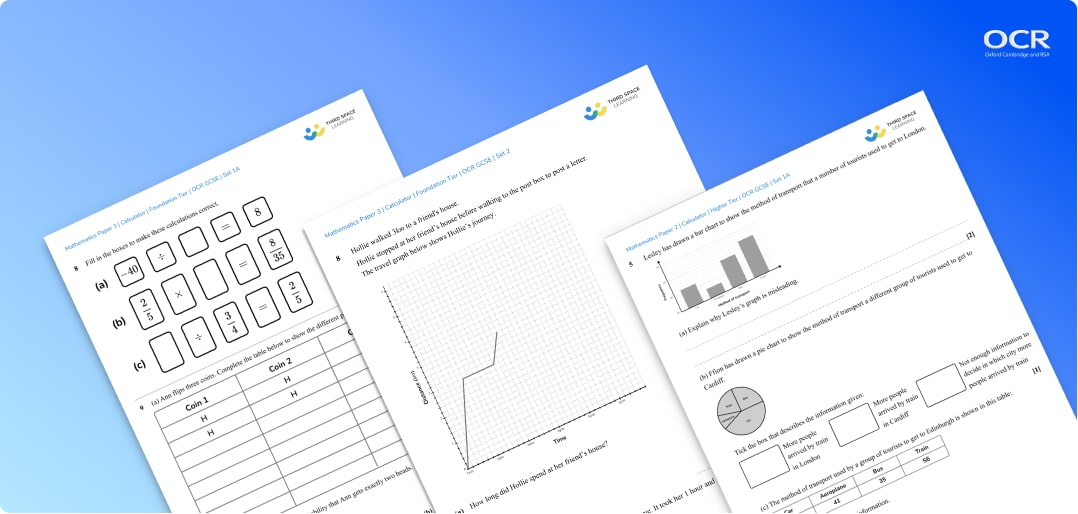What can you expect from the GCSE maths OCR papers?

Exam questions are set out in the style of the sample assessment materials from the exam boards and OCR past papers (Foundation tier & Higher tier). All align with the OCR maths course and include answers and mark schemes.

Set 1a of the maths exam papers are based on the GCSE mathematics advanced information 2022.

Sets 2 onwards (more to come) are predicted papers based on GCSE maths past papers and the relevant OCR specification for each tier.

All maths questions on the exam papers are created by current or former OCR examiners and expert maths teachers.

## How to use these OCR maths papers

OCR Foundation Papers include:

OCR GCSE Maths Foundation Paper 1 (non-calculator)

OCR GCSE Maths Foundation Paper 2 (calculator)

OCR GCSE Maths Foundation Paper 3 (calculator)

OCR Higher Papers include:

OCR GCSE Maths Higher Paper 1 (non-calculator)

OCR GCSE Maths Higher Paper 2 (calculator)

OCR GCSE Maths Higher Paper 3 (calculator)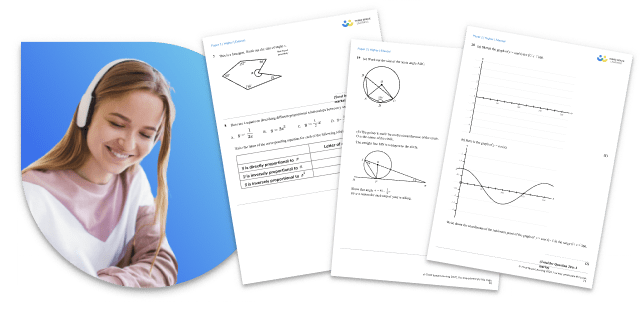These specially created OCR maths papers are an essential addition to your GCSE maths revision for GCSE exams.

They closely match the OCR GCSE maths past papers provided by the exam board and in relation to the grade boundaries we know them to be fairly accurate predicted papers.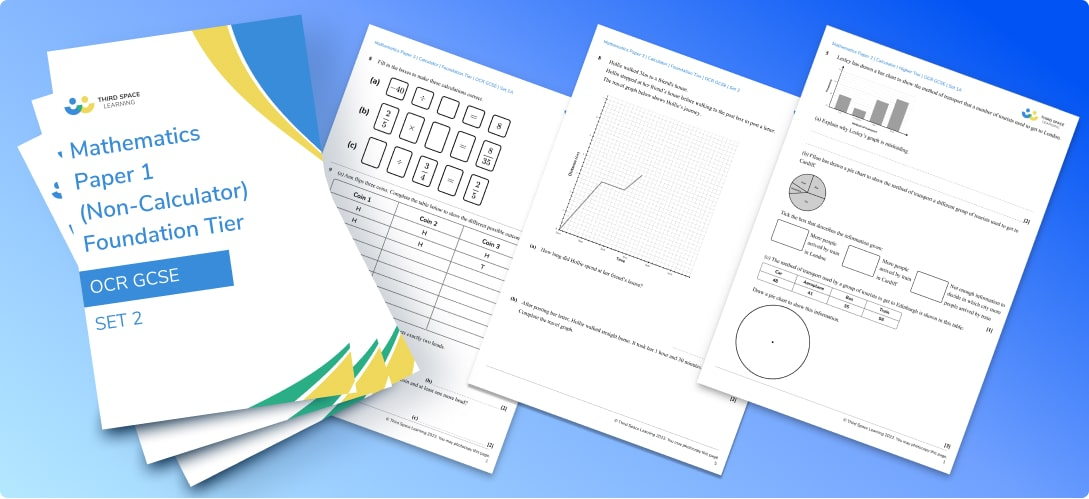While the style and layout of these question papers will be familiar to students the content is new and unique.

Schools who follow the OCR GCSE mathematics syllabus often use these practice papers for mock exams or as an assessment resource - the mark scheme and topic coverage enable you also to see individual gaps for each student.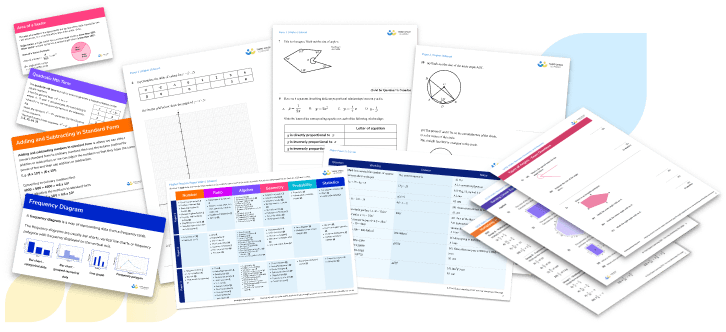If you need further GCSE maths exam practice and preparation take a look at the free worksheets, diagnostic quizzes, and GCSE revision resources available below.

As well as these OCR maths papers we also provide free practice papers based on AQA Maths Papers & Past Papers and Edexcel Maths Papers & Past Papers (all of which are broadly suitable as part of revision for the IGCSE exam too).

More GCSE Maths Papers

GCSE Maths Papers & Past Papers

GCSE Maths Higher Papers

GCSE Maths Foundation Papers

For old spec papers and the AQA GCSE maths, OCR GCSE maths and Edexcel GCSE maths past papers follow these links:

Edexcel

AQA

OCR

## Can't find what you're looking for?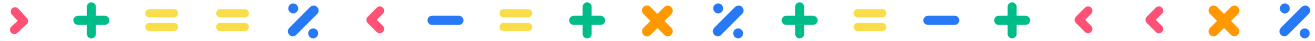## Teaching support from the UK’s largest provider of in-school maths tuition

At Third Space Learning, we’re on a mission to help teachers like you shape your students into confident, able mathematicians.

Request a quote